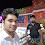How to calculate the cement, sand quantity for plastering

# How to calculate the cement, sand quantity for plasteringIn this construction video tutorial provides some step-by-step guidelines to how to calculate the cement sand quantity for plastering.
Let us suppose,
Mortar ratio = 1:6 where 1 is cement and 6 part is sand.
Plaster thickness = 12 mm = 0.012 m
Cement density = 1440 kg/m3
1 m3 = 35.3147 ft3
Suggested Plaster Thickness
12 mm for internal walls or brick wall plain surface
15 mm for 4” or 9” brick wall rough surface 20 mm for two plaster layers normally utilized for external walls
Mortar Wet volume = 1 x 1 x 0.012 = 0.012 m3
Mortar Dry volume = 0.012 x 1.30 = 0.0156 m3
Mortar Dry volume (sand + cement) = 30 – 35 % greater than wet volume = wet volume x 1.30
Concrete Dry volume (aggregate + sand + cement) = wet volume x 1.54
As we know that mortar ratio = 1:6, from which 1 + 6 = 7 = sum of ratio
Amount of cement in plaster = 1/7 x 0.0156 x 1440 = 3.21 kg
Also in bags = 3.21/50 = 0.064 bag = approximately 1 bag
Amount of sand in plaster = 6/7 x 0.0156 = 0.0133 m3
Sand quantity in cubic feet = 0.0133 x 35.3147 = 0.47 ft3

Continue Reading about the "How to calculate the cement, sand quantity for plastering" on the next page below

1.2.I need this book could you send this for me ghulamhaidararyan1370@gmail.com

3.need this book could you send this for me
najmularch@gmail.com

4.5.Jdelima59@gmail.com

6.very informative kindly email the book to harry.anyanzo@gmail.com

7.Sir can u send me PDF of this book

8.I need a training on this engineering society, i want to be a member.

9.Sir forward the important details to me through.... Champmarine106@gmail.com.

10.Best design for construction work.thanks